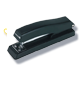# Medical Coding Books

AAPC's ICD-10, HCPCS Level II, Procedural Coding Expert and CPT® medical code books are the best in the industry for the best value. Get all the codes, descriptions, guidelines, annotations, reference tables, colored illustrations, coding tips and clinical examples you need in a color-coded, tabbed, spiral–bound format you'll love—specially designed for speed and accuracy.

• Developed by coders, for coders
• Earn AAPC CEUs with book purchases
• Expanded Quick View Flow Charts in ICD-10-CM
• Exclusive monthly code update newsletter
• Exclusive AAPC coding tips
• Highlighted MACRA codes
• Adhesive tabs decrease look-up time
• Color symbols, alerts and illustrations
• Spiral-bound (lay flat for easy use)
• Best value for your money

2021
$119.99$79.99
2021
$359.99$229.99
2021
$119.99$79.99
2021
$121.95$117.99
2021
$359.99$139.99
2021
$119.99$79.99
2021
$359.99$229.99
2021
$239.99$119.99
2021
$479.99$259.99
2021
$119.99$69.99
2021
$159.99$69.99
2021
$239.99$119.99
2021
$749.99$599.99
2021
$639.99$479.99
2021
$819.99$719.99
2021
$749.99$629.99
2022
$359.99$229.99
2022
$119.99$79.99
2022
$359.99$139.99
2022
$243.90$199.99
2022
$359.99$229.99
2022
$119.99$79.99
2022
$119.99$69.99
2022
$239.99$119.99
2022
$479.99$259.99
2022
$121.95$116.99
2022
$119.99$79.99
2022
$159.99$69.99
2022
$359.99$229.99
2022
$119.99$79.99
2022
$239.99$119.99
2022
$121.95$116.99
2021
$199.99$129.99
through July 31
2021
$199.99$129.99
through July 31
2021
$199.99$129.99
through July 31
2021
$199.99$129.99
through July 31
2021
$299.99$169.99
through July 31
2021
$199.99$129.99
through July 31
2021
$199.99$129.99
through July 31
2021
$199.99$129.99
through July 31
2021
$199.99$129.99
through July 31
2021
$199.99$129.99
through July 31
2021
$199.99$129.99
through July 31
2021
$299.99$169.99
through July 31
2021
$199.99$129.99
through July 31
2021
$199.99$129.99
through July 31
2021
$199.99$129.99
through July 31
2021
$199.99$129.99
through July 31
2021
$199.99$129.99
through July 31
2021
$199.99$129.99
through July 31
2021
$199.99$129.99
through July 31
2021
$199.99$129.99
through July 31
2021
$199.99$129.99
through July 31
2021
$199.99$129.99
through July 31
2021
$359.99$229.99
2021
$121.95$117.99
2021
$119.99$79.99
2021
$119.99$79.99
2021
$119.99$79.99
2021
$119.99$69.99
2021
$159.99$69.99
2021
$359.99$139.99
2021
$479.99$259.99
2021
$239.99$119.99
2021
$243.90$199.99
2021
$239.98$99.99
2021
$239.98$99.99
2021
$239.98$99.99
2021
$239.98$99.99
2021
$319.98$99.99
2021
$719.98$329.99
2021
$719.98$199.99
2021
$959.98$399.99
2021
$479.98$169.99
2022
$119.99$79.99
2022
$119.99$79.99
2022
$119.99$69.99
2022
$159.99$69.99
2022
$359.99$139.99
2022
$239.99$119.99
2022
$479.99$259.99
2022
$239.98$99.99
2022
$239.98$99.99
2022
$239.98$99.99
2022
$239.98$99.99
2022
$319.98$99.99
2022
$719.98$329.99
2022
$359.99$199.99
2022
$479.98$169.99
2022
$959.98$379.99
2022
$749.99$599.99
2022
$639.99$479.99
2022
$749.99$629.99
2022
$819.99$719.99
2021
$199.99$119.99
2021
$199.99$119.99
2021
$199.99$119.99
2021
$199.99$119.99
2021
$199.99$119.99
2021
$299.99$139.99
2021
$399.98$119.99
2021
$199.99$119.99
2021
$199.99$119.99
2021
$199.99$119.99
2021
$199.99$119.99
2021
$299.99$139.99
2021
$199.99$119.99
2021
$199.99$119.99
2021
$199.99$119.99
2021
$199.99$119.99
2021
$199.99$119.99
2021
$199.99$119.99
2021
$199.99$119.99
2021
$199.99$119.99
2021
$199.99$119.99
2021
$199.99$119.99
2021
$399.98$199.99
2021
$399.98$199.99
2021
$399.98$199.99
2021
$399.98$199.99
2021
$399.98$199.99
2021
$599.98$249.99
2021
$199.99$199.99
2021
$399.98$199.99
2021
$399.98$199.99
2021
$399.98$199.99
2021
$399.98$199.99
2021
$599.98$249.99
2021
$399.98$199.99
2021
$399.98$199.99
2021
$399.98$199.99
2021
$399.98$199.99
2021
$399.98$199.99
2021
$399.98$199.99
2021
$399.98$199.99
2021
$399.98$199.99
2021
$399.98$199.99
2021
$399.98$199.99
Second Edition
$149.99$129.99
Second Edition
$119.99$99.99
Second Edition
$169.99$149.99
Second Edition
$169.99$149.99
Second Edition
$209.99$189.99
Second Edition
$169.99$149.99
Second Edition
$209.99$189.99
Second Edition
$189.99$169.99
Second Edition
$169.99$149.99
Second Edition
$169.99$149.99
Second Edition
$149.99$129.99
Second Edition
$119.99$99.99
Second Edition
$169.99$149.99
Second Edition
$169.99$149.99
Second Edition
$209.99$189.99
Second Edition
$169.99$149.99
Second Edition
$209.99$189.99
Second Edition
$189.99$169.99
Second Edition
$169.99$149.99
Second Edition
$169.99$149.99
Second Edition
$359.98$149.99
Second Edition
$299.98$119.99
Second Edition
$399.98$169.99
Second Edition
$399.98$169.99
Second Edition
$479.98$209.99
Second Edition
$399.98$169.99
Second Edition
$479.98$209.99
Second Edition
$439.98$189.99
Second Edition
$399.98$169.99
Second Edition
$399.98$169.99
2022
$199.99$189.99
2022
$199.99$189.99
2022
$199.99$189.99
2022
$199.99$189.99
2022
$199.99$189.99
2022
$299.99$229.99
2022
$199.99$189.99
2022
$199.99$189.99
2022
$199.99$189.99
2022
$199.99$189.99
2022
$199.99$189.99
2022
$299.99$229.99
2022
$199.99$189.99
2022
$199.99$189.99
2022
$199.99$189.99
2022
$199.99$189.99
2022
$199.99$189.99
2022
$199.99$189.99
2022
$199.99$189.99
2022
$199.99$189.99
2022
$199.99$189.99
2022
$199.99$189.99
2022
$199.99$119.99
2022
$199.99$119.99
2022
$199.99$119.99
2022
$199.99$119.99
2022
$199.99$119.99
2022
$299.99$139.99
2022
$399.98$119.99
2022
$199.99$119.99
2022
$199.99$119.99
2022
$199.99$119.99
2022
$199.99$119.99
2022
$299.99$139.99
2022
$199.99$119.99
2022
$199.99$119.99
2022
$199.99$119.99
2022
$199.99$119.99
2022
$199.99$119.99
2022
$199.99$119.99
2022
$199.99$119.99
2022
$199.99$119.99
2022
$199.99$119.99
2022
$199.99$119.99
2022
$399.98$199.99
2022
$399.98$199.99
2022
$399.98$199.99
2022
$399.98$199.99
2022
$399.98$199.99
2022
$599.98$249.99
2022
$199.99$199.99
2022
$399.98$199.99
2022
$399.98$199.99
2022
$399.98$199.99
2022
$399.98$199.99
2022
$599.98$249.99
2022
$399.98$199.99
2022
$399.98$199.99
2022
$399.98$199.99
2022
$399.98$199.99
2022
$399.98$199.99
2022
$399.98$199.99
2022
$399.98$199.99
2022
$399.98$199.99
2022
$399.98$199.99
2022
$399.98$199.99

## The AAPC Difference

AAPC's ICD-10-CM Expert, HCPCS Level II Expert, Procedural Coding Expert, and Procedure Desk Reference medical code books offer the accuracy and efficiency you need with features you want. Official code sets and guidelines by CMS and AMA© paired with AAPC features, such as detailed descriptions, comprehensive annotations, integrated reference tables, precise colored illustrations, numerous coding tips and clinical examples in a color-coded, tabbed, spiral–bound format provide the best value."This book has a lot of helpful information right at the coder’s fingertips, and that is what we need—quick reference materials. Time is of the essence."
Lisa O, CPC"I LOVE THIS BOOK!!! The instructions are concise. The rationale is explained in the simplest terms. This is very well written. It’s easy to read and easy to understand… almost to good to be true!"
Mary W. CPC, CEMC

Why AAPC?

• Tools that benefit both students and experienced coders
• MACRA focused icons, sections and tips included
• New adhesive tabs decreasing look-up time in every book
• Spiral-binding lays flat to save space
• Illustrations, color symbols, and alerts
• Exam Help throughout ICD-10-CM and ICD-10-PCS books
• Exclusive AAPC website content throughout the year to keep you up-to-date on changes
• Bundled with AAPC Code Change Webinars